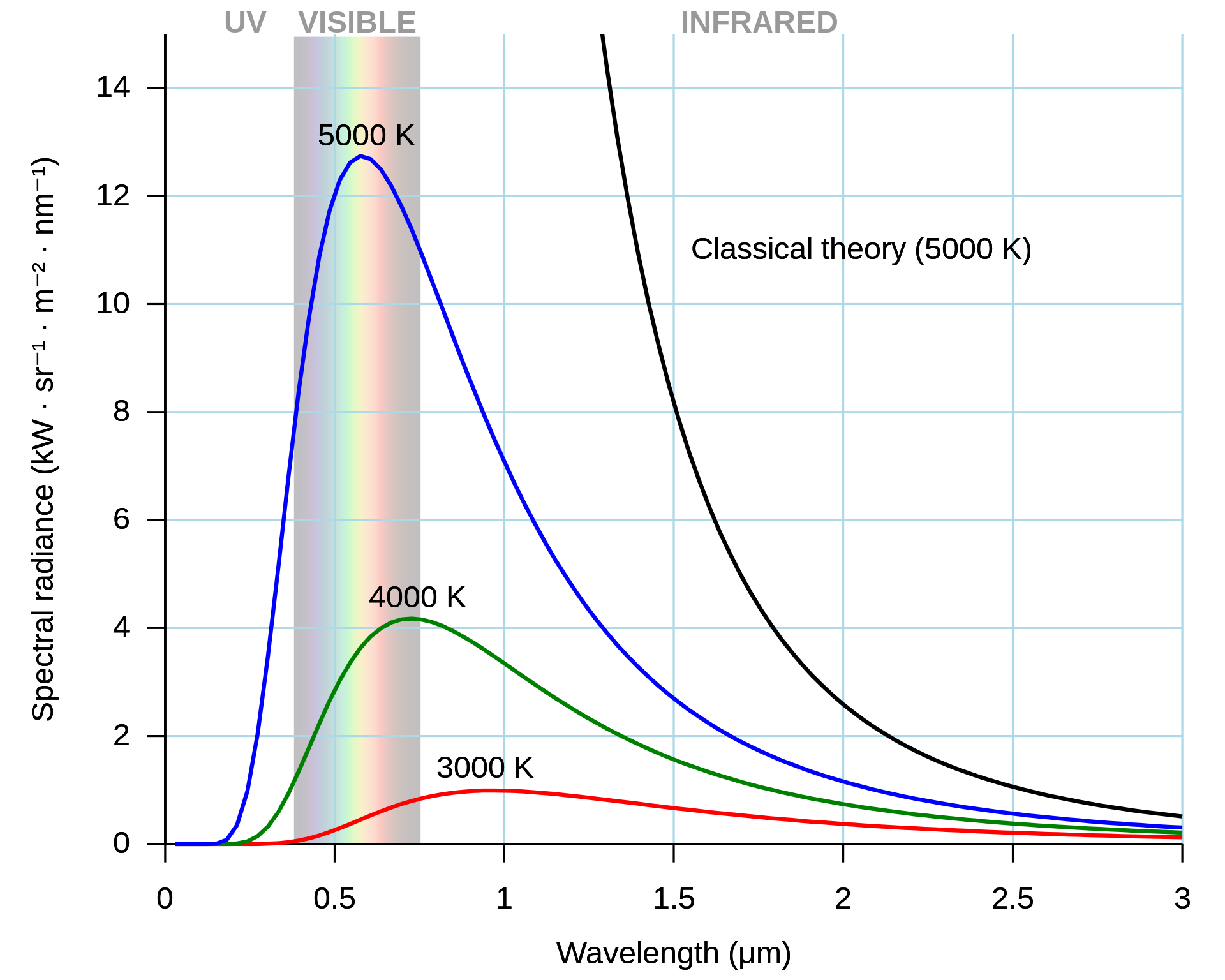## Ultraviolet Catastrophe

### Wein (1889)

$$E=\sigma T^4$$, Stefan-Boltzman Law. $$\sigma=5.67\cdot10^8$$. $$U(\nu,T)=A\nu^3\exp(-\beta\nu/T)$$### Planck (1900)

Oscillators $$\nu$$ are quantized by energy such that $$E=n\hbar\nu$$, $$n\in\mathbb{N}$$.

• $$U(\nu,T)=\frac{8\pi\nu^2}{c^3}\frac{\hbar\nu}{\exp(\hbar\nu/(k_BT))-1}$$

Created: 2023-06-25 Sun 02:28

Validate